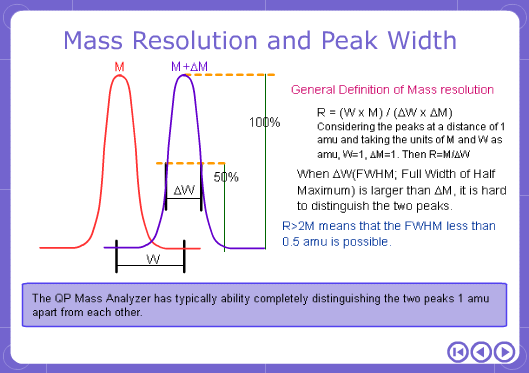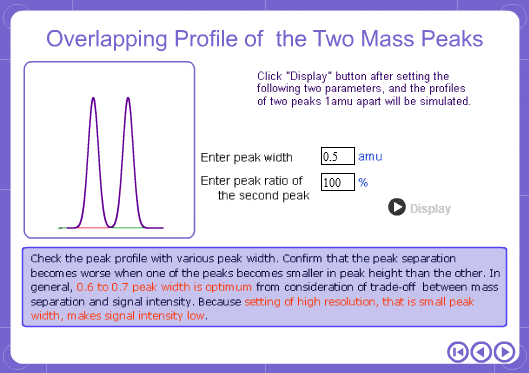# With regard to resolution; What does R > 2M mean? Specifically, just how good is R > 2M?

## 1. BASIC MASS SPECTROMETRY

### 04. With regard to resolution; What does R > 2M mean? Specifically, just how good is R > 2M?

The M in 2M stands for mass number. The resolution of QPMS is proportional to mass and this type of expression is therefore used. A resolution of 2M means the resolution would be 200 when the mass number is 100 and 600 when the mass number is 300. In addition, with QPMS the resolution is calculated using the peak half-height width (width at 1/2 peak height). FWHM stands for width at half height.

* A more detailed explanation follows. Assume the peak profile shown in the following page is obtained.